Courses

# Kinetic Theory Of Gases MCQ Level – 2 (part - 1)

## 10 Questions MCQ Test Topic wise Tests for IIT JAM Physics | Kinetic Theory Of Gases MCQ Level – 2 (part - 1)

Description
This mock test of Kinetic Theory Of Gases MCQ Level – 2 (part - 1) for Physics helps you for every Physics entrance exam. This contains 10 Multiple Choice Questions for Physics Kinetic Theory Of Gases MCQ Level – 2 (part - 1) (mcq) to study with solutions a complete question bank. The solved questions answers in this Kinetic Theory Of Gases MCQ Level – 2 (part - 1) quiz give you a good mix of easy questions and tough questions. Physics students definitely take this Kinetic Theory Of Gases MCQ Level – 2 (part - 1) exercise for a better result in the exam. You can find other Kinetic Theory Of Gases MCQ Level – 2 (part - 1) extra questions, long questions & short questions for Physics on EduRev as well by searching above.
QUESTION: 1

### The temperature at which the average speed of H2 equals that of O2 at 320K. Select one:

Solution: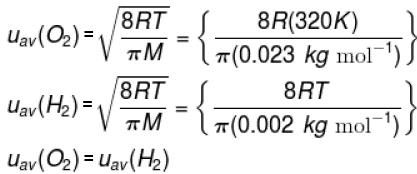Therefore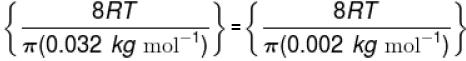which gives,   T = 20 K
The correct answer is: 20 K

QUESTION: 2

### The critical constant for water are 647 K, 22.09 MPa and 0.0566 dm3 mol-1, the value of a and b are respectively. Select one:

Solution:

We have
Tc = 647 K
pc = 22.09 MPa = 22.09 × 103 kPa
Vc = 0.0566 dm-3 mol-1
Thus,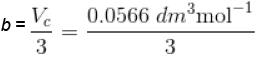= 0.0189 dm3 mol-1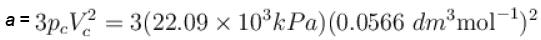= 212.3 kPa dm6 mol-2
The correct answer is:  = 212.3 kPa dm6 mol-2, 0.0189 dm3 mol-1

QUESTION: 3

### Calculate molecular diameter d of helium from its van der Waals constant b = 24 cm3 mol-1 Select one:

Solution:

Since, b = 4 × volume occupied by the molecule in 1 mole of a gas
or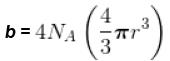therefore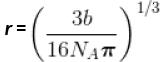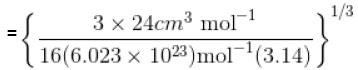= 1.335 × 10-8 cm = 133.5 pm
d = 2r = 267 pm
The correct answer is: 267 pm

QUESTION: 4

The kinetic energy of 0.5 mol of an ideal gas at 273 K
Select one:

Solution:

Total kinetic energy;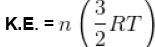K.E. = (0.5 mol)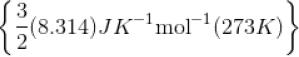= 1702 J
The correct answer is: 1702 J

QUESTION: 5

The mean free path of the molecule of a certain gas at 300K is 2.6×10-5m. The collision diameter of the molecule is 0.26 nm then the number of molecule per unit volume of the gas.
Select one:

Solution:

Here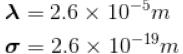T = 300K
Since,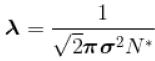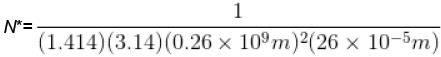= 1.281 × 1023 m-3
The correct answer is: 1.281 × 1023 m-3

QUESTION: 6

Using vander Waals equation, the pressure exerted by 22g of carbon dioxide in 0.5 dm3 at 298.15 K is (Given : a = 363.76 kPa dm6 mol-2 and b = 42.67 cm3 mol-1 )
Select one:

Solution:

Vander Waals equation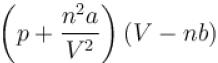= nRT
and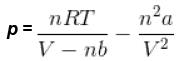So,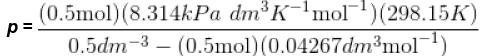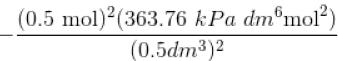= 2589.31 kPa – 363.76 kPa
= 2225.55 kPa.
The correct answer is: 2222.55 kPa

QUESTION: 7

Calculate the fraction of N2 molecule at 101.325 kPa and 300 K whose speed are in the range of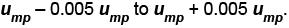Select one:

Solution:

Most probable speed,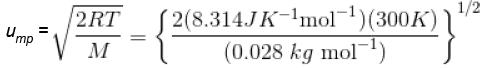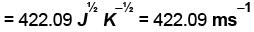Since,  0.005 ump = (0.005) (422.09 ms-1)
= 2.11 ms-1
Therefore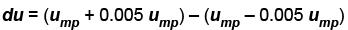4.22 ms-1
According to Maxwell distribution law,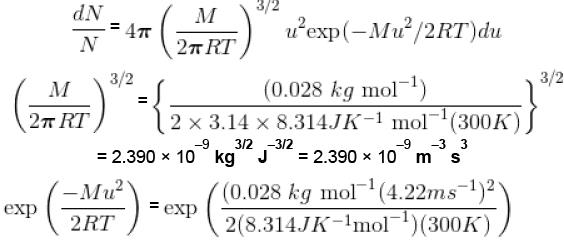= exp (–1.000) = 0.3679
Hence,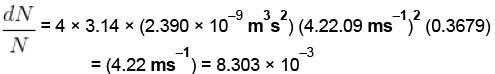The correct answer is: 8.303 × 10-3

QUESTION: 8

The average speed of H2 molecules, if the density of the gas at 101.325 kPa is 0.09gdm-3
Select one:

Solution:

Density ρ = 009 gdm-3 = 0.09 kgm-3
Average speed,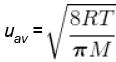Also,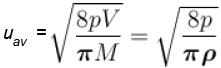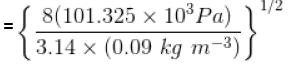= 1694 ms-1
The correct answer is: 1694 ms-1

QUESTION: 9

What is the ratio of the number of molecule having speed in the range of 2ump and 2ump + du to the number of molecule having speeds in the range of ump + du.
Select one:

Solution:

If dN1 is the number of molecule in the speed range ump to ump + du and dN2 is the corresponding number in the speed range 2ump to 2ump + du, then according to the Maxwell distribution, we have,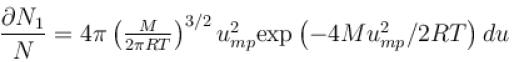and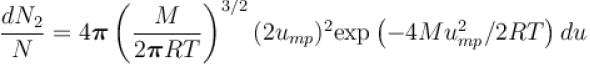therefore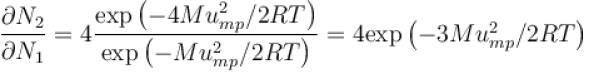Now, Since,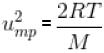therefore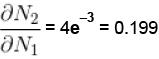QUESTION: 10

A bulb of capacity 1 dm3 contain 1.03 × 1023 gaseous hydrogen molecules and the pressure exerted by these molecules is 101.325 kPa. Calculate the average square molecular speed.
Select one:

Solution:

We have
V = 1dm3
N = 1.3 × 1023
p = 101.325 kPa
We known, number of moles
n = N/NA
n =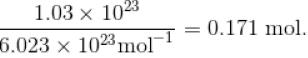T = pV/nR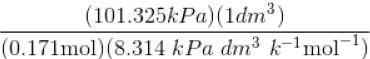= 71.27 K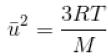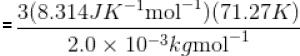= 8.888 × 10 5Jkg-1
= 8.888 × 105 (ms-1 )2
The correct answer is: 8.888 × 105 (ms-1 )2